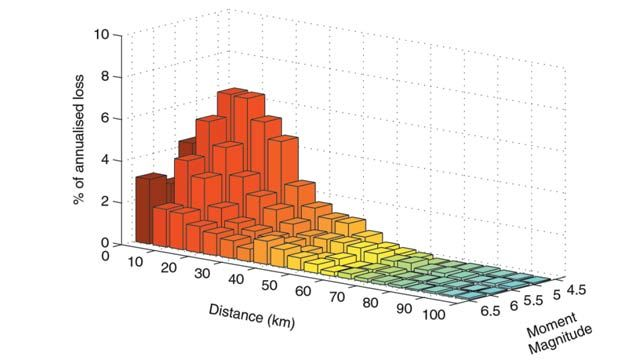User Stories

# Geoscience Australia Saves \$50,000 in Development Costs by Using MATLAB

## Challenge

To better understand earthquakes and their potential impact on populated areas

## Solution

Perform data processing and simulations in MATLAB to estimate earthquake hazards and their associated risks

## Results

• \$50,000 in development costs saved
• Earthquake hazard results generated in just one day
• Up to 75% less code to write

“MATLAB enabled us to perform all our modeling and produce diagnostic plots simultaneously. This would have been much more difficult in traditional programming languages."

David Robinson, Geoscience AustraliaEarthquake hazard model developed in MATLAB.

On December 28, 1989, an earthquake measuring 5.6 on the Richter scale occurred near Newcastle, Australia, damaging nearly 50,000 buildings. Geoscience Australia (GA) subsequently set out to help Newcastle and surrounding areas gain a better understanding of earthquake risk and reduce their potential impact.

Using MATLAB, and collaborating with Aon Impact Forecasting, GA integrated earthquake hazard assessment and risk models with details about buildings in the Newcastle and Lake Macquarie area to produce the most detailed earthquake risk assessment ever published in Australia. MATLAB enabled GA to develop the models quickly and efficiently.

"We cut the time for modeling and simulating earthquake hazards from 20.7 hours to a little over three hours with the JIT-Accelerator and some clever vectorization in MATLAB," says David Robinson, geophysicist for the Minerals and Geohazard Division at GA.

## Challenge

Geoscience Australia’a earthquake analysis involved estimating earthquake hazard and evaluating earthquake risk. They estimated building damage and economic loss for a subsample of the buildings in Newcastle and Lake Macquarie and then extrapolated the results to all buildings in the region.

Evaluating earthquake hazard was a spatial problem that involved estimating, or modeling, the level of ground motion expected within a specific time period across a geographical region. For this work, GA set up a grid of points and modeled the hazard at each of those points.

The hazard was modeled by simulating earthquakes of varying magnitude, attenuating the associated energy to the grid points, and amplifying the energy as it interacted with local geology.

Geoscience Australia worked with large data sets. The simulated data for the earthquake hazard model represented roughly 13,000 sites and 12,500 simulated earthquakes. The earthquake risk model represented a sample of 6,000 buildings and 1,200 simulated earthquakes.

## Solution

Building on existing MATLAB applications, the GA team wrote the vulnerability code and used MATLAB to simulate local ground shaking, calculate building damage, and predict the effect of the simulated earthquake on surrounding rock and soil. “Having the ability to streamline tasks within one integrated environment was very beneficial,” Robinson says. They used historical and geological information about previous earthquakes to constrain the probability of future earthquakes in terms of magnitude and location.

“MATLAB lets us handle large data sets and perform calculations efficiently and quickly,” says Robinson. “Using the built-in MATLAB functions, it was easy to sort and find the required data from within very large matrices. The new JIT-Accelerator and some clever programming in MATLAB enabled us to perform routines more than six times faster.”

For earthquake hazard models, the team used MATLAB routines to sort through the matrices to identify events of interest and estimate the hazard. To estimate the building damage, they used functions in MATLAB to efficiently calculate the maximum building displacement and acceleration for each of the 6,000 buildings in the database. They used this data to calculate the probabilities of being in a given damage state. They then calculated the economic damage for each building by aggregating the dollar loss for all buildings in Newcastle for a specified period.

Geoscience Australia now has models that define the probability of a future earthquake, define the levels of ground shaking in relation to the distance from the earthquake, and its expected impacts. Based on this data, they have made recommendations to the Earthquake Loading Standards Committee of Australia, which is responsible for developing building codes that will help protect new buildings and key facilities, such as emergency services and hospitals, from earthquake damage.

## Results

• \$50,000 in development costs saved. “The development of this model has taken roughly six months with two people working full-time,” Robinson explains. “Using MATLAB instead of C cut our development time by approximately twelve months. Considering the average employee cost minus the cost of two MATLAB licenses, we yielded a saving of approximately \$50,000 US or \$90,000 AUD.”

• Earthquake hazard results generated in just one day. "By using the new version of MATLAB with the JIT-Accelerator and some clever programming, we were able to provide tested earthquake hazard results using a number of submodel combinations within one working day. Previously, it would have taken two to three days to provide results for one combination of submodels,” Robinson says.

• Up to 75% less code to write. “Because MATLAB is a high-level language, we have a variety of prewritten functions at our fingertips, dramatically reducing the development time,” explains Robinson. “There is much less code to write — with some models having 75% fewer lines of code than C.”